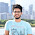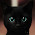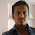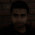## Sunday, 10 February 2013

### Random Number Generator in Verilog | FPGA

In verilog a random number can be generated by using the \$random keyword but that works only for simulation purposes and cannot be implemented. So when we need a random number for implementation i.e. in hardware it can be achieved using an LFSR (Liner Feedback Shift Register). An LFSR is simply a shift register with some of its bits (known as taps) XOR'd with themselves to create a feedback term.

When implementing an LFSR it's width and it's repeatability must be kept under consideration .An N-bit LFSR will be able to generate (2**N) - 1 random bits before it starts repeating. For example a 30 bit LFSR will have 1073741823 random states before repeating, so for most practical purposes this can be considered true random.

In an LFSR the MSB will always be the feedback point also the main thing to take care of while coding an LFSR is to know which bits are the taps (to be selected for XOR). This is confusing as the taps are different for different size registers. For example an 8 bit LFSR will have its 8th, 6th, 5th and 4th bits XOR'd with each other whereas a 10 bit LFSR will have its 10th and 7th bits XOR'd to create the random numbers. So clearly there is no pattern to this, but thankfully Xilinx has calculated all of this and made it available to us in its online documentation. The table below shows the tap locations for LFSR's upto 168 bits.

An LFSR is good for generating a stream of random bits. It does not generate a random number by itself but only the feedback bit is random. However that does not mean that we cannot use this fact to generate a random number. In my code below I have an output of 13 bits and a 13 bit random number is accomplished by shifting the feedback bit enough times to equal the desired output size. In my case when it has shifted 13 times it will be assigned to the output. This way every bit is random thus making the whole number pretty random if you ask me.

Below is a code for a 13 bit LFSR. Notice my selection of taps is taken from the table above:

```module LFSR (
input clock,
input reset,
output [12:0] rnd
);

wire feedback = random ^ random ^ random ^ random;

reg [12:0] random, random_next, random_done;
reg [3:0] count, count_next; //to keep track of the shifts

always @ (posedge clock or posedge reset)
begin
if (reset)
begin
random <= 13'hF; //An LFSR cannot have an all 0 state, thus reset to FF
count <= 0;
end

else
begin
random <= random_next;
count <= count_next;
end
end

always @ (*)
begin
random_next = random; //default state stays the same
count_next = count;

random_next = {random[11:0], feedback}; //shift left the xor'd every posedge clock
count_next = count + 1;

if (count == 13)
begin
count = 0;
random_done = random; //assign the random number to output after 13 shifts
end

end

assign rnd = random_done;

endmodule```

As requested, below is the test bench for the above code, not much stimulus in it, its just a simple reset.
```module test;

// Inputs
reg clock;
reg reset;

// Outputs
wire [12:0] rnd;

// Instantiate the Unit Under Test (UUT)
LFSR uut (
.clock(clock),
.reset(reset),
.rnd(rnd)
);

initial begin
clock = 0;
forever
#50 clock = ~clock;
end

initial begin
// Initialize Inputs

reset = 0;

// Wait 100 ns for global reset to finish
#100;
reset = 1;
#200;
reset = 0;
// Add stimulus here

end

initial begin
\$display("clock rnd");
\$monitor("%b,%b", clock, rnd);
end
endmodule```

Do note that this is not pure random, this number can be predicted if the algorithm is known. Thus don't use this for security purposes ;)

1.how do we show its output on a FPGA board??

1.I coded this solely to be used as a module within my reaction tester. But if you want to use this as your main module on your FPGA board you will have to multiplex it to the seven segment display. More on it here: http://simplefpga.blogspot.co.uk/2012/07/seven-segment-led-multiplexing-circuit.html

An example and further reading here: http://simplefpga.blogspot.co.uk/2012/07/00-to-99-two-digit-decimal-counter-via.html

2.Hi. you have explained the code very clearly and nicely. I would really appreciate it if you could explain the verilog code for a bipartite lfsr as well.. Thanx.
Regards.

3.This comment has been removed by a blog administrator.

4.I'm running this program in Xylinx software but its showing some error while running the RTL schematic. Otherwise the syntax check shows no error.

5.Hi. I'm getting this error while simulating the above program code
"this signal is connected to multiple drivers".
Can you help me sort out this problem.

1.I got this problem too, did you solved this out?

2.The same. There is a bug in the code. There are multiple drivers for signal count - in both always clauses. There should be a different code enclosed in single always() clause.

3.Can you elaborate on what you mean by "different code"? Thanks

6.always @ (*)
begin
random_next = random; //default state stays the same
count_next = count;

random_next = {random[11:0], feedback}; //shift left the xor'd every posedge clock
count_next = count + 1;

- See more at: http://simplefpga.blogspot.in/2013/02/random-number-generator-in-verilog-fpga.html#sthash.DHLvWMA1.dpuf

7.I cant Understand That Things,,

8.A synthesizable code for 10bit Random Number Generator

each 10 clocks a new random value is generated

///////////////
`resetall
`timescale 1ns/10ps
module rndm_gen (
input clock,
input reset,
output reg [9:0] rnd
);

wire feedback;
wire [9:0] lfsr_next;

//An LFSR cannot have an all 0 state, thus reset to non-zero value
reg [9:0] reset_value = 13;
reg [9:0] lfsr;
reg [3:0] count;

// pragma translate_off
integer f;
initial begin
f = \$fopen("output.txt","w");
end
// pragma translate_on

always @ (posedge clock or posedge reset)
begin
if (reset) begin
lfsr <= reset_value;
count <= 4'hF;
rnd <= 0;
end
else begin
lfsr <= lfsr_next;
count <= count + 1;
// a new random value is ready
if (count == 4'd9) begin
count <= 0;
rnd <= lfsr; //assign the lfsr number to output after 10 shifts
// pragma translate_off
\$fwrite(f,"%0d\n",rnd);
// pragma translate_on
end
end
end

// X10+x7
assign feedback = lfsr ^ lfsr;
assign lfsr_next = {lfsr[8:0], feedback};

// pragma translate_off
always @ (*) begin
if (rnd == reset_value) begin
\$fclose(f);
\$finish();
end
end
// pragma translate_on
endmodule

// pragma translate_off
module rndm_tb;
wire clock;
wire reset;
wire [9:0] rnd;

reg clk;
reg rst;

rndm_gen rando(
.clock (clock),
.reset (reset),
.rnd (rnd)
);

assign clock = clk;
assign reset = rst;

// generate the clock
initial begin
clk <= 0;
forever begin
#10 ;
clk <= ~clk;
end
end

initial begin
rst <= 1;
#50;
rst <= 0;
end

endmodule
// pragma translate_on
////////////////

9.its not working for 16 bit

1.As mentioned this is "a synthesizable code for 10bit" you should modify it to work for 16bits. Take care of the conditions bits and the taps locations

2.it has worked finally.. can you please upload the schematic for 16 bit?

10.Can you give the code after debugging it?
The error is: Signal count in unit LFSR is connected to following multiple drivers: